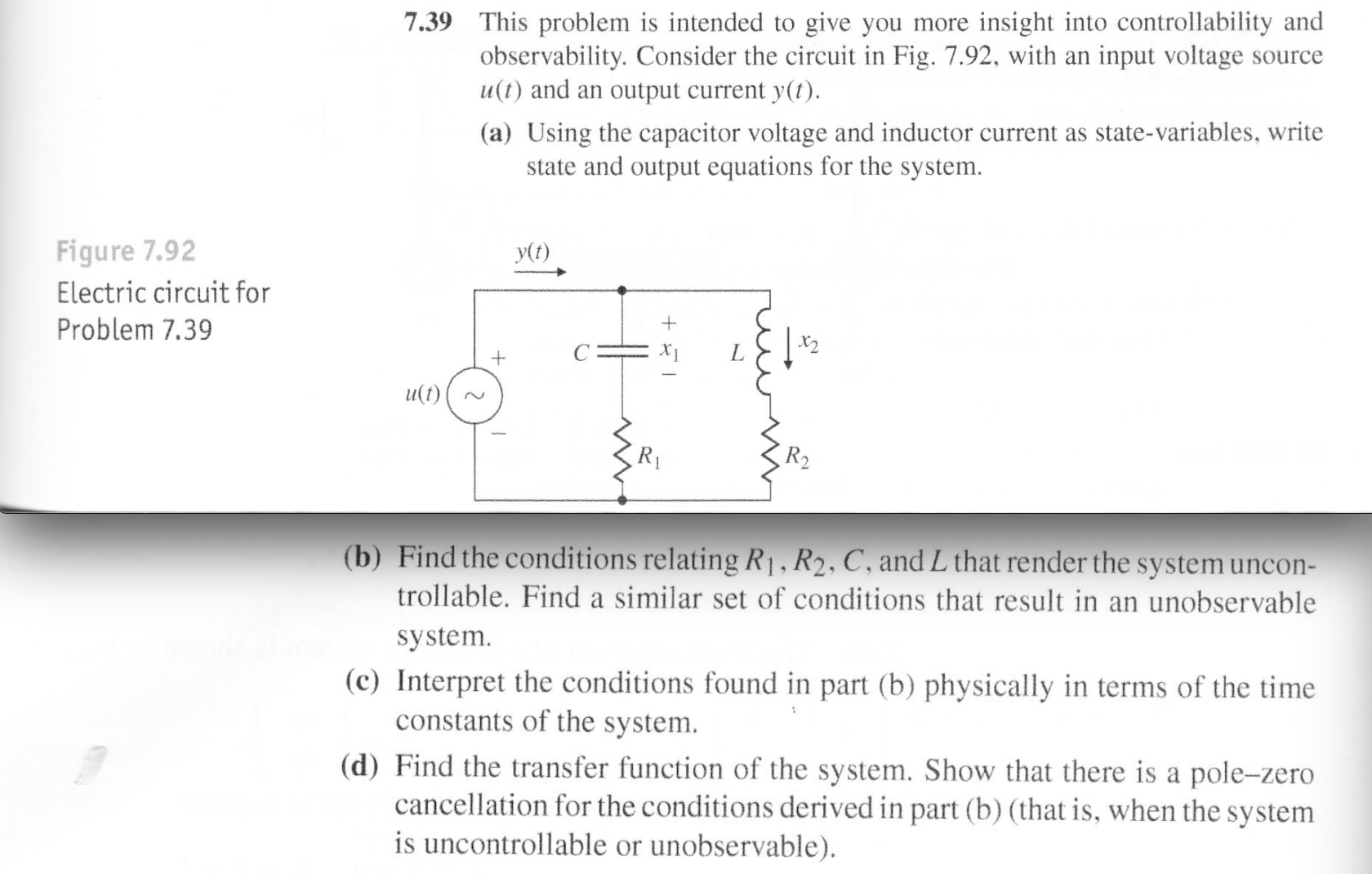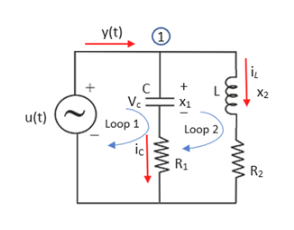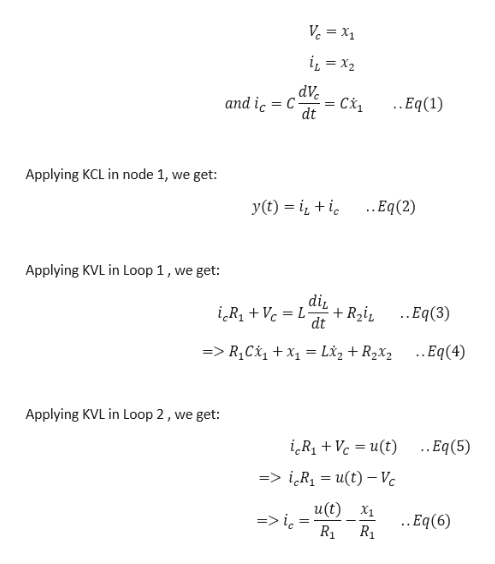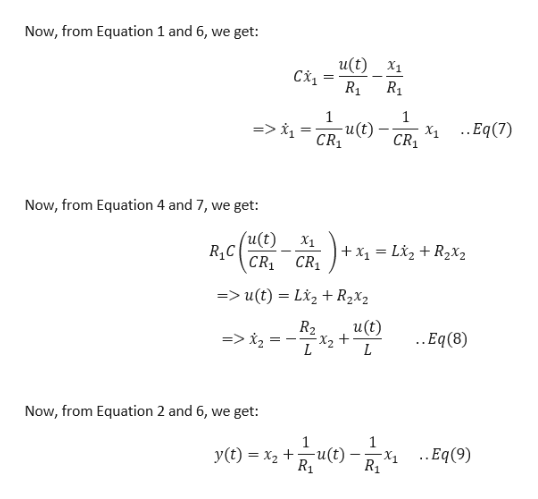Question

Feedback Control of Dynamic Systems (7th edition) - Problem 7.39help_outlineImage Transcriptionclose7.39 This problem is intended to give you more insight into controllability and observability. Consider the circuit in Fig. 7.92, with an input voltage u(t) and an output current y(t). Source (a) Using the capacitor voltage and inductor current as state-variables, write state and output equations for the system Figure 7.92 y(t) Electric circuit for Problem 7.39 + u(t) R2 (b) Find the conditions relating R1, R2, C, and L that render the system uncon- trollable. Find a similar set of conditions that result in an unobservable system (c) Interpret the conditions found in part (b) physically in terms of the time constants of the system (d) Find the transfer function of the system. Show that there is a pole-zero cancellation for the conditions derived in part (b) (that is, when the system is uncontrollable or unobservable) fullscreen
Step 1

Hi there!! Since there are four parts in the question, I will be answering only the first two parts. In case you need the third and fourth part, you need to repost the complete question and specifically ask for the solution of the third and fourth part. Thankyou.

The circuit diagram is shown below:help_outlineImage Transcriptionclosey(t) Ve X1 u(t) Loop 1 Loop 2 R1 R2 wWM fullscreen
Step 2

Let us say, from the given circuit :help_outlineImage TranscriptioncloseVe = x1 dVeCi dt ..Eq(1) and ic C Applying KCL in node 1, we get: ..Eq(2) y(t) Applying KVL in Loop 1, we get: di ..Eq(3) + R2i dt iRVL- =>R1C1xL*z +R2x2 ..Eq(4) Applying KVL in Loop 2, we get: .Eq(5) iRiVc ut) => iR1 = u(t) - Vc u(t) X1 ..Eq(6) fullscreen
Step 3

Further,...help_outlineImage TranscriptioncloseNow, from Equation 1 and 6, we get: u(t) X1 1 -u(t)- CR1 => i CR X1.Eq(7) Now, from Equation 4 and 7, we get: (u(t) X1 X1L2+R2X2 CR1 CR1 => u(t) = L*2 + R2x2 u(t) R2 => i2= ..Eq(8) L Now, from Equation 2 and 6, we get: ..Eq(9) u(t) y(t) x2 + fullscreen

Want to see the full answer?

See Solution

Want to see this answer and more?

Our solutions are written by experts, many with advanced degrees, and available 24/7

See Solution
Tagged in

Electrical Engineering